Courses

# NCERT Solutions(Part- 6)- Mensuration Class 8 Notes | EduRev

## Class 8 : NCERT Solutions(Part- 6)- Mensuration Class 8 Notes | EduRev

The document NCERT Solutions(Part- 6)- Mensuration Class 8 Notes | EduRev is a part of the Class 8 Course Class 8 Mathematics by VP Classes.
All you need of Class 8 at this link: Class 8

VOLUME OF CUBE, CUBOID AND CYLINDER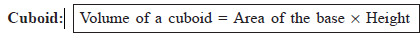TRY THESE
Question: Find the volume of the following cuboids.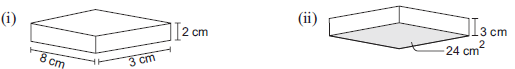Solution: (i) Base area = 8 cm * 3 cm
Height = 2 cm
∴ Volume of the cuboid = (8 cm * 3 cm) * 2 cm = 48 cm3
(ii) Base area = 24 m2
Height = 3 cm
∴ Volume of the cuboid = Base area * height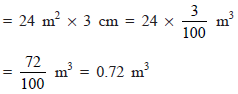Cube: Cube is a special case of a cuboid such that its Length = Breadth = Height
∴ Its volume = Edgs * Edge * Edge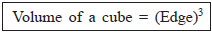Question: Find the volume of the following cubes (a) with a side 4 cm and (b) with a side 1.5 m.
Solution: (a) Side (edge) of the cube = 4 cm
∴ Volume of the cube = (Edge)3 = (4 cm)3
= 4 * 4 * 4 cm3 = 64 cm3
(b) Side (edge) of the cube = 1.5 m
∴ Volume of the cube = (Edge)3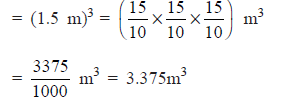SOLUTION TO THINK, DISCUSS AND WRITE
Question: A company sells biscuits. For packing purpose they are using cuboidal boxes; box A → 3 cm * 8 cm * 20 cm, box B → 4 cm * 12 cm * 10 cm. What size of the box will be economical for the company? Why? Can you suggest any other size (dimensions) which has the same volume but is more economical than these?
Solution: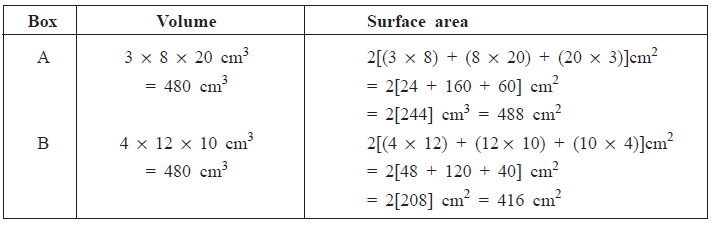Clearly, Volume of box B = Volume of box A, but its surface area is less, so its more economical
for the company.
Another size: 8 cm * 6 cm * 10 cm
∵ Volume = 8 * 6 * 10 cm3 = 480 cm3
Surface area = 2[(8 * 6) + (6 * 10) + (8 * 10)] cm2
= 2[48 + 60 + 80] cm2
= 2 cm2 = 376 cm2
Since its surface area is still less than the box B.
∴ It is more economical for the company.
Cylinder: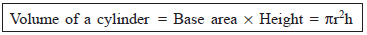TRY THESE
Question: Find the volume of the following cylinders.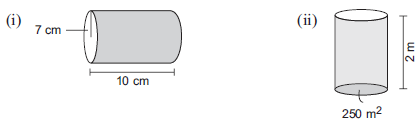Solution: (i) Radius (r) = 7 cm
Height (h) = 10 cm
∴ Volume of the cylinder = πr2h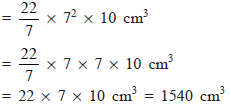(ii) Base area = 250 m2
Height = 2 m
∴ Volume of the cylinder = Base area * Height
= 250 m2 * 2 m = 500 m3

REMEMBER
(i) 1 cm= 1 mL
(ii) 1 litre = 1000 cm
(iii) 1 m3 = 1000000 cm = 1000 mL

EXERCISE 11.4
Question 1. Given a cylindrical tank, in which situation will you find surface area and in which situation volume.
(a) To find how much it can hold.
(b) Number of cement bags required to plaster it.
(c) To find the number of smaller tanks that can be filled with water from it.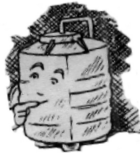Solution: (a) Volume (b) Surface area (c) Volume

Question 2. Diameter of cylinder A is 7 cm, and the height is 14 cm. Diameter of cylinder B is 14 cm and height is 7 cm. Without doing any calculations can you suggest whose volume is greater? Verify it by finding the volume of both the cylinders. Check whether the cylinder with greater volume also has greater surface area?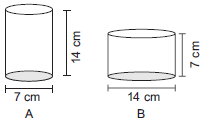Solution: In volume of a cylinder, the radius is multiplied by squaring it.
∴ Volume of cylinder B will be more than that of A.
Volume of cylinder A
Radius (r)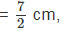Height (h) = 14 cm
∴ Volume of a cylinder A = πr2h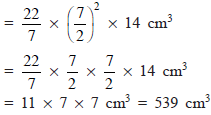Volume of cylinder B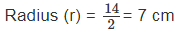Height (h) = 7 cm
∴ Volume of the cylinder B = πr2h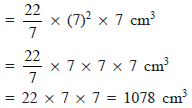Thus, cylinder B has greater volume.
Now,
Surface area of cylinder A = 2 * π * r * (r + h)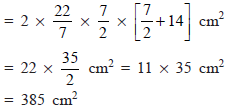Surface area of cylinder B = 2πrh(r + h) =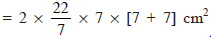= 2 * 22 *  cm2
= 616 cm2
Thus, the cylinder of greater capacity has greater (more) surface area.

Question 3. Find the height of a cuboid whose base area is 180 cm2 and volume is 900 cm3.
Solution: Let the height of the cuboid = h cm
∴ Base area * Height = Volume
or                 180 * h = 900
or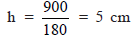Hence, the required height of the cuboid = 5 cm

Question 4. A cuboid is of dimensions 60 cm * 54 cm * 30 cm. How many small cubes with side 6 cm can be placed in the given cuboid?
Solution: Volume of the cuboid = 60 cm * 54 cm * 30 cm
= (60 * 54 * 30) cm3
Volume of the small cube = (6 * 6 * 6) cm3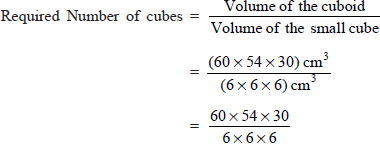= 10 * 9 * 5 = 450
Thus, 450 small cubes can be placed in the given cuboid.

Question 5. Find the height of the cylinder whose volume is 1.54 mand diameter of the base is 140 cm.
Solution: Diameter = 140 cm ⇒ Radius (r)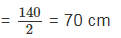Let height of the cylinder be h m.
∴ Volume = πr2h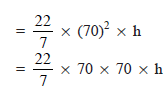Since, volume of the cylinder is 1.54 m3.
∴ 22 * 10 * 70 * h = 1.54 * 1000000 = 1540000
or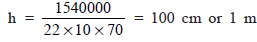Question 6. A milk tank is in the form of cylinder whose radius is 1.5 m and length is 7 m. Find the quantity of milk in litres
that can be stored in the tank?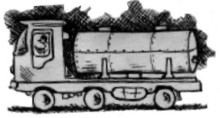Solution: Radius = 1.5 m =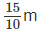Height = 7 m
∴ Volume of the tank = πr2h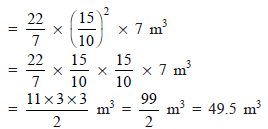∵ 1 m= 1000 litres
∴ Quantily of milk in the tank = 49.5 x 1000 litres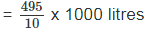Question 7. If each of a cube is doubled,
(i) How many times will its surface area increase?
(ii) How many times will its volume increase?
Solution: Let the original edge = x
∴ Increased edge = 2x
(i) ∴ Original S.A. = 6x2
Increased S.A. = 6(2x)2 = 24x2
Since,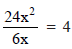∴ Surface area is increased by 4 times.

(ii) Original volume = x3
Increased volume = (2x)= 8x3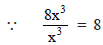∴ Volume is increased by 8 times.

Question 8. Water is pouring into a cuboidal reservoir at the rate of 60 litres per minute. If the volume of reservoir is 108 m3, find the number of hours it will take to fill the reservoir.
Solution: Volume of the reservoir = 108 m3
∵                                           1 m3 = 1000 litres
∴            Capacity of the reservoir = 108 * 1000 litres
= 108000 litres
Amount of water poured in 1 minute = 60 litres
∴ Amount of water to be poured in 1 hour = 60 * 60 litres
Thus, number of hours required to fill the reservoir =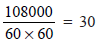∴ The required number of hours = 30

Offer running on EduRev: Apply code STAYHOME200 to get INR 200 off on our premium plan EduRev Infinity!

89 docs|16 tests

,

,

,

,

,

,

,

,

,

,

,

,

,

,

,

,

,

,

,

,

,

;